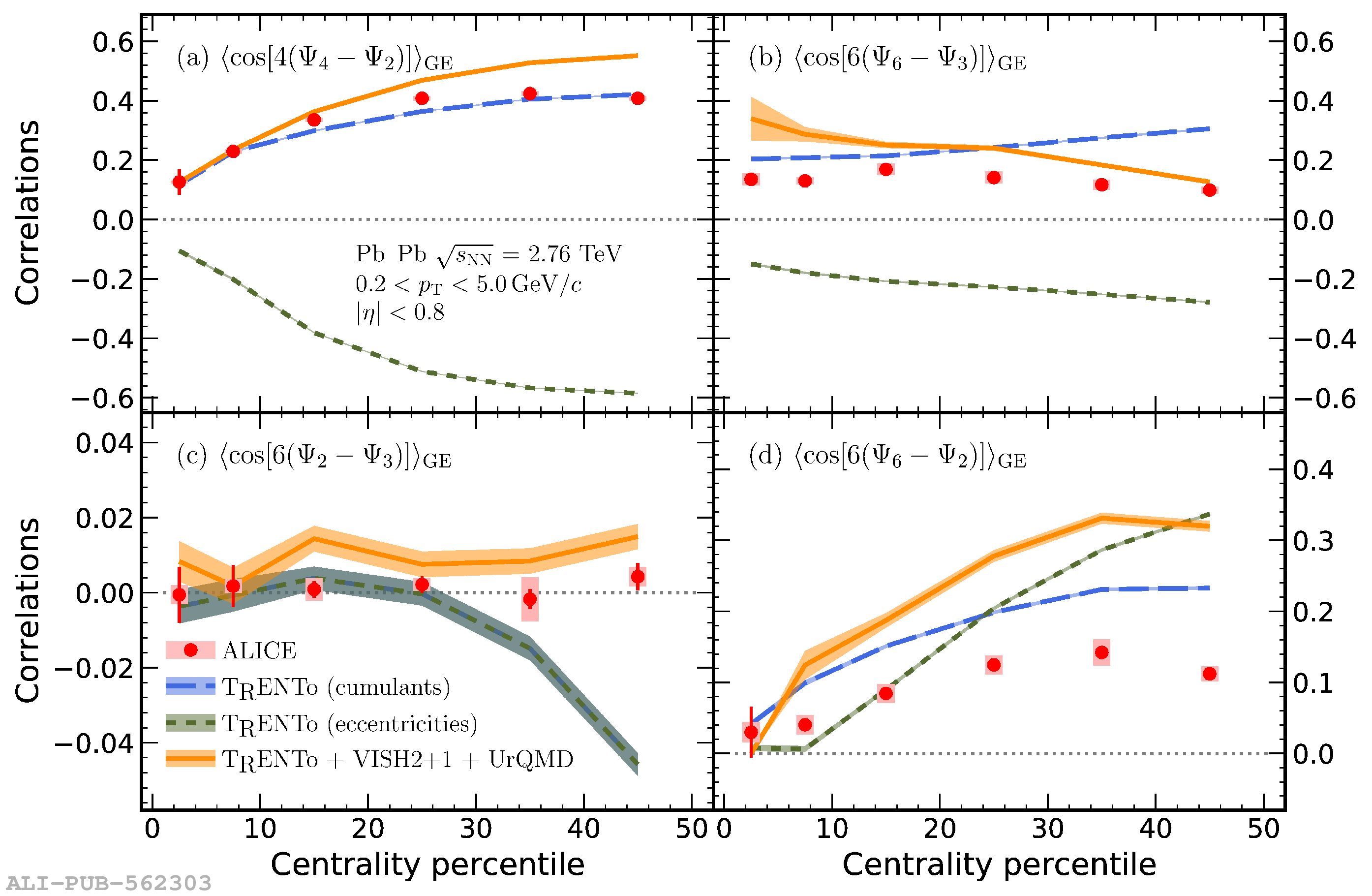# Figure 4

 Experimental data for correlations between two symmetry planes compared to theoretical predictions in the initial and final state obtained with T$_\text{R}$ENTo and T$_\text{R}$ENTo+VISH(2+1)+UrQMD, respectively. For $\langle\cos\left[6\left(\Psi_2-\Psi_3\right)\right]\rangle_\text{GE}$ (c), the initial state predictions calculated via eccentricities and energy density cumulants, $\langle \cos [6(\phi_2-\phi_3)]\rangle_{\text{GE}}$ and $\langle \cos[6(\Phi_2-\Phi_3)]\rangle_{\text{GE}}$, fully overlap. Statistical (systematic) uncertainties of the ALICE data are shown as lines (boxes). The statistical uncertainties of the models are represented by the colored bands.